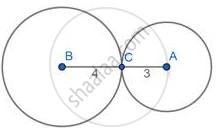# If Two Circles with Diameters 8 Cm and 6 Cm Respectively Touch Externally, Find the Distance Between Their Centers. - Geometry

Diagram

If two circles with diameters 8 cm and 6 cm respectively touch externally, find the distance between their centers.

#### Solution

AB = 4cm and BC=3cmIf two circles touch externally, then the distance between their centers is the sum of their radii.

distance (AC) = 4+3 = 7cm.

The distance between the centers= 7cm

Concept: Touching Circles
Is there an error in this question or solution?